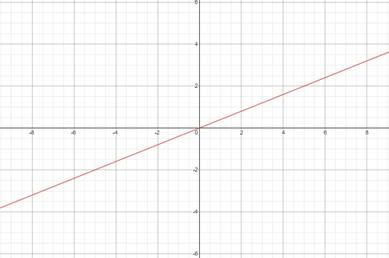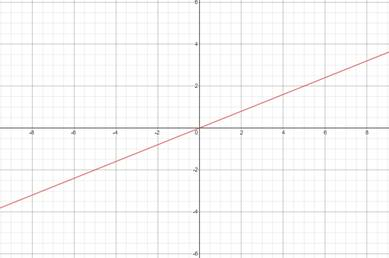# Slope and y-intercept of given equation, plot its graph### Precalculus: Mathematics for Calcu...

6th Edition
Stewart + 5 others
Publisher: Cengage Learning
ISBN: 9780840068071### Precalculus: Mathematics for Calcu...

6th Edition
Stewart + 5 others
Publisher: Cengage Learning
ISBN: 9780840068071

#### Solutions

Chapter 1.10, Problem 48E
To determine

## Slope and y-intercept of given equation, plot its graph

Expert Solution

Slope=25yintercept=0### Explanation of Solution

Given information:

2x5y=0

Formula used:

Slope-intercept form of line: y=mx+c , where m is the slope and c is the y-intercept cut by the line.

2x5y=0

Converting the line into slope intercept form

2x5y=05y=2xy=25x+0

m=25c=0

Plotting the Graph

Finding two points on line

x=02(0)5y=05y=0y=0

(0,0)

y=22x5(2)=02x=10x=5

(5,2)### Have a homework question?

Subscribe to bartleby learn! Ask subject matter experts 30 homework questions each month. Plus, you’ll have access to millions of step-by-step textbook answers!